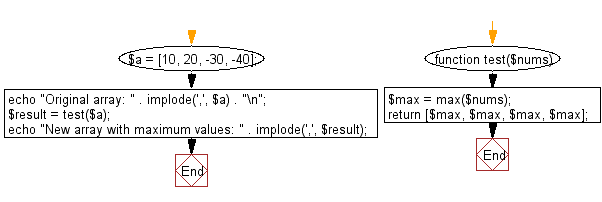﻿ PHP Exercises: Find out the maximum element between the first or last element in a given array of integers, replace all elements with maximum element - w3resource# PHP Exercises: Find out the maximum element between the first or last element in a given array of integers, replace all elements with maximum element

## PHP Basic Algorithm: Exercise-92 with Solution

Write a PHP program to find out the maximum element between the first or last element in a given array of integers ( length 4), replace all elements with maximum element.

Sample Solution:

PHP Code :

``````<?php
function test(\$nums)
{
\$max = max(\$nums);

return [\$max, \$max, \$max, \$max];

}

\$a = [10, 20, -30, -40];

echo "Original array: " . implode(',', \$a) . "\n";

\$result = test(\$a);

echo "New array with maximum values: " . implode(',', \$result);
``````

Sample Output:

```Original array: 10,20,-30,-40
New array with maximum values: 20,20,20,20
```

Flowchart:PHP Code Editor:

What is the difficulty level of this exercise?

﻿

## PHP: Tips of the Day

\$_REQUEST: This SuperGlobal Variable is used to collect data submitted by a HTML Form

Example:

This code save in a php file and run in the browser.

```<!DOCTYPE html>
<html>
<body>

<form method="post" action="<?php echo \$_SERVER['PHP_SELF'];?>">
NAME: <input type="text" name="user">
<button type="submit">SUBMIT</button>
</form>
<?php
if (\$_SERVER["REQUEST_METHOD"] == "POST") {
\$name = htmlspecialchars(\$_REQUEST['user']);
if(empty(\$name)){
echo "Name is empty";
} else {
echo \$name;
}
}
?>
</body>
</html>
```

Output:

```Owen
```